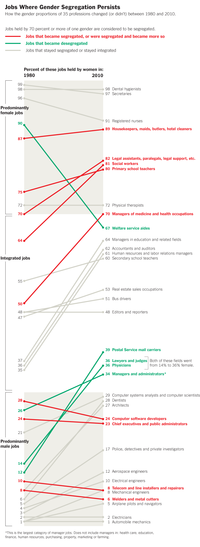The New York Times has an excellent staff that produces visually interesting graphics for the general public. However, because their graphs need to be understood by all Times readers, the staff sometimes creates a complicated infographic when a simpler statistical graph would show the data in a clearer manner.

A recent graphic was discussed by Kaiser Fung in his article, "When Simple Is too Simple." Kaiser argued that the Times made a poor choice of colors in a graphic (shown at right) that depicts the proportion of women in certain jobs and how that proportion has changed between 1980 and 2010.

I agree with Kaiser that the colors should change, and I will discuss colors in a subsequent blog post. However, I think that the graph itself suffers from some design problems. First, it is difficult to see overall trends and relationships in the data. Second, in order to understand the points on the left side of the graph, you have to follow a line to the right side of the graph in order to find the label. Third, the graph is too tall. At a scale in which you can read the labels, only half the graph appears on my computer monitor. If printed on a standard piece of paper, the labels would be quite small.

You can overcome these problems by redesigning the graph as a scatter plot. I presume that the Times staff rejected the scatter plot design because they felt it would not be easily interpretable by a general Times reader. Another problem, as we will see, is that a scatter plot requires shorter labels that the ones that are used in the Times graphic.

A scatter plot of the same data is shown in the next figure. (Click to enlarge.) The graph shows the proportion of women in 35 job categories. The horizontal axis shows the proportion in 1980 and the vertical axis shows the proportion in 2010. Jobs such as secretary, hygienist, nurse, and housekeeper are primarily held by women (both in 1980 and today) and appear in the upper right. Jobs such as auto mechanic, electrician, pilot, and welder are primarily held by men (both in 1980 and today) and appear in the lower left. Jobs shown in the middle of the graph (bus driver, reporter, and real estate agents) are held equally by men and women.

The diagonal line shows the 1980 baseline. Points that are displayed above that line are jobs for which the proportion of women has increased between 1980 and 2010. This graph clearly shows that the proportion of women in most jobs has increased or stayed the same since 1980. (I classify a deviation of a few percentage points as "staying the same," because this is the margin of error for most surveys.) Only the job of "welfare aide worker" has seen a substantial decline in the proportion of women.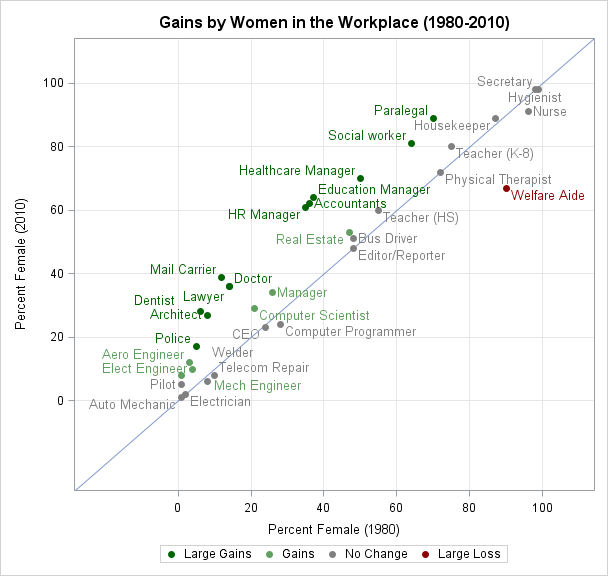The markers are colored on a red-green scale according to the gains made by women. Large gains (more than 10%) are colored a dark green. Lesser gains (between 5% and 10% are a lighter green. Similarly, jobs for which the proportion of women declined are shown in red. Small changes are colored gray.

This graph shows the trend more clearly than the Times graphic. You can see at a glance that women have made strides in workplace equity across many fields. In many high-paying jobs such as doctor, lawyer, dentist, and various managerial positions, women have made double-digit gains.

The shortcomings of the graph include using shorter labels for the job categories, and losing some of the ability to know the exact percentages in each category. For example, what is the proportion of females that work as a welfare aide in 2010? Is it 68%, 67%, or 66%? This static graph does not give the answer, although if the graph is intended for an online display you can create tooltips for each point.

For those readers who are interested in the details, you can download the SAS program that creates this plot. The program uses three noteworthy techniques:
1. A user-defined SAS format is used to display the differences in proportions into a categorical variable with levels "Large Gains," "Gains," "No Change," and so on.
2. An attribute map is used to map each category to a specified shade of red, gray, and green. This feature was introduced in SAS 9.3. My next blog post will discuss this step in more detail.
3. The DATALABEL= option is used to specify a variable that should be used to label each point. The labels are arranged automatically. The SAS research and development staff spent a lot of time researching algorithms for the automatic placement of labels, and for this example the default algorithm does an excellent job of placing each label near its marker while avoiding overlap between labels.

What do you think? Which graphic would you prefer to use to examine the gains made by women in the workplace? Do you think that the New York Times readers are sophisticated enough to read a scatter plot, or should scatter plots be reserved for scientific communication?Sometimes it is useful to group observations based on the values of some variable. Common schemes for grouping include binning and using quantiles.

In the binning approach, a variable is divided into k equal intervals, called bins, and each observation is assigned to a bin. In this scheme, the size of the groups is proportional to the density of the grouping variable. In the SAS/IML language, you can use the BIN function to assign observations to bins.

However, sometimes it is useful to have approximately the same number of observations in each group. In this case, you can group the observations into k quantiles. One way to do this is to use PROC RANK and to specify the GROUPS= option, as follows:

```%let NumGroups = 4; proc rank data=Sashelp.class out=class groups=&NumGroups ties=high; var Height; /* variable on which to group */ ranks Group; /* name of variable to contain groups 0,1,...,k-1 */ run;```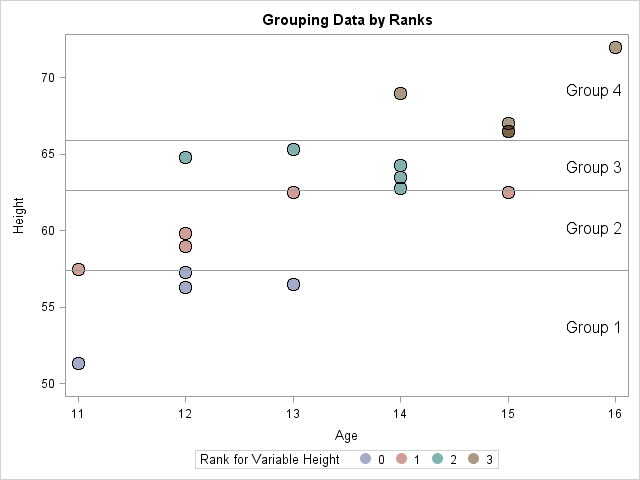A scatter plot of the data (click to enlarge) is shown with markers colored by group membership. There are 19 observations in this data set. The RANK procedure puts four observations into the first group and five observations into each of the next three groups, which is an excellent distribution of the 19 observations into four groups. (In Group 4, there are two markers with the coordinates (15, 66.5).) The TIES= option specifies how to handle tied values. I specified TIES=HIGH because that option is consistent with the definition of a quantile for a discrete distribution.

In the SAS/IML language, you can use the QNTL subroutine and the BIN function to construct a function that groups observations by quantiles, as follows:

```proc iml; /* Assign group to each observation based on quantiles. For example, g=GroupRanks(x,4) assigns each observation to a quartile. Assume x is a column vector and k is less than the number of unique values of x */ start GroupRanks(x, k); if k<2 then return(j(nrow(x), 1, 1)); dx = 1/k; p = do(dx, 1-dx/2, dx); /* 4 groups ==> p={0.25 0.50 0.75} */ call qntl(q, x, p); Group = bin(x, .M // q // .I); return(Group); finish;   /* run example */ use Sashelp.Class; read all var {Height}; close; Group = GroupRanks(Height, 4);```

The call to the GroupRanks function results in the same assignment of observations to groups as PROC RANK, except that the GroupRanks function uses the identifiers 1,2,...,k. The GroupRanks function uses two tricks:

• The QNTL function finds k–1 "cut points" that divide the values of x into k groups, with about the same number of observations in each group.
• The special missing values .M and .I correspond to "minus infinity" and "plus infinity," respectively. These special values are appended to the other cut points and supplied as parameters to the BIN function. The BIN function returns a vector with the values 1, 2, ...,k that classifies each observation into a bin.

There are several statistical uses for creating k groups with roughly the same number of observations in each group. One is when assigning colors to a choropleth map or a heat map. Rather than chop the range of the response variable into k equal intervals, it is often better to choose 5 or 7 quantiles and color the regions by quantiles of the response. This technique is especially valuable when the response variable has a long-tailed distribution.With the US presidential election looming, all eyes are on the Electoral College. In the presidential election, each state gets as many votes in the Electoral College as it has representatives in both congressional houses. (The District of Columbia also gets three electors.) Because every state has two senators, it is the number of representatives in the House that determines how many electors are awarded to each state.

The 2012 election is the first presidential election since the 2010 census and subsequent reapportionment of representatives. Usually the apportionment of the Electoral College is represented as a static choropleth map that show the number of electors by state at any instance in time. Recently, however, the US Census Bureau's Data Visualization Gallery published a visualization of "Ranks of States by Congressional Representation" over time. This graphic is reproduced below (click to enlarge):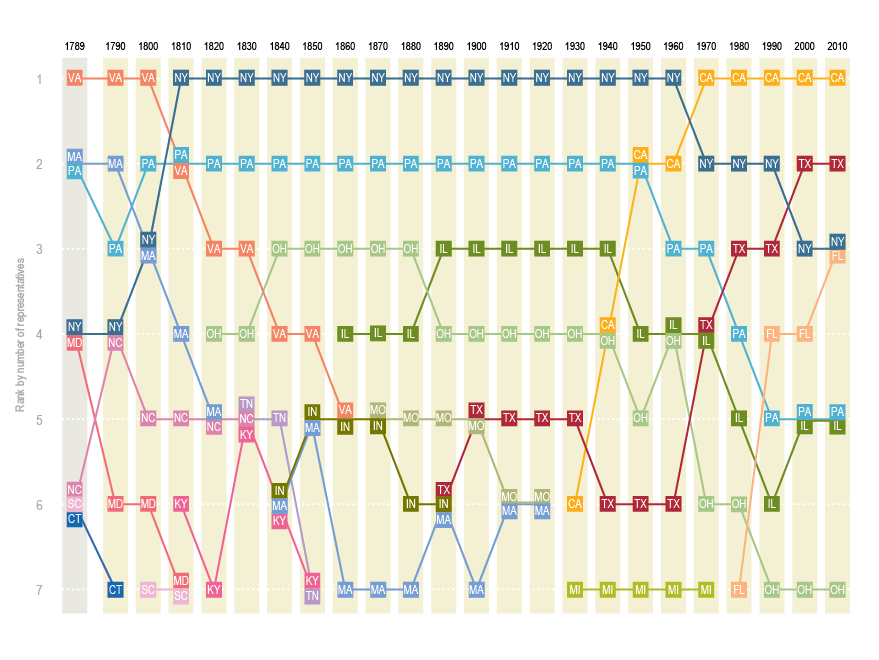The graph shows the rank of the top seven most populous states after each US census. You can see a few interesting facts such as:

• New York and Pennsylvania have always been among the most populous states.
• California, which became a state in 1850, popped onto the list in 1930 and rapidly became the most populous state.
• Texas, which became a state in 1845, entered the list in 1890 and is now the second most populous state.
• Florida, which became a state in 1845, broke into the top seven in 1980 and currently has the same number of representatives as New York.
• Virginia and Massachusetts were once among the populous states, but have since dropped off the list.

The reason that the US Census Bureau used ranks on the vertical scale is because the number of representatives has changed over the years: from 106 in 1790, to 243 at the time of the Civil War, to the modern value of 435, which was adopted in 1960. It occurred to me that it might be more interesting to see the percentage of the representatives that were apportioned to each state throughout the US history. I also thought the graph might look better if states don't "pop on" and "pop off" the way that Tennessee and Michigan do. I would prefer to see a state's entire history or representation.

With that goal in mind, I downloaded the data from the Census Bureau in CSV format. Instead of computing the ranks of the states for each decade, I grouped the states into quintiles according to their current number of representatives. Thus there are five groups, where each group contains 10 states. The first quintile contains the least populous states (Wyoming, Vermont, and so forth) and the fifth quintile contains the most populous states (California, New York, and so forth). The time series plot of the most populous states follows. The label for Ohio is partially hidden under the label for Georgia.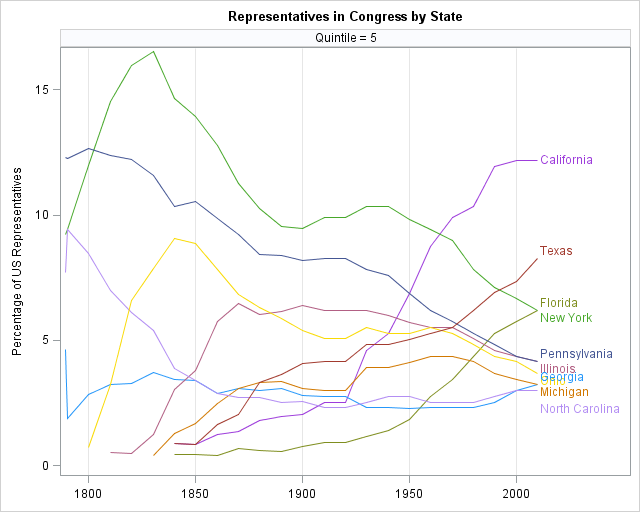You can download the complete SAS program that manipulates, rearranges, and plots the data. The program produces plots for all 50 states. I tried different approaches, such as assigning states into quintiles according to the historical average of the representation percentage, but I the graph that I show is the one that compares the best with the US Census graph. The graph is different than a graph that shows the population of each state over time, because this graph shows relative growth rather than absolute growth.Robert Allison posted a map that shows the average commute times for major US cities, along with the proportion of the commute that is attributed to traffic jams and other congestion. The data are from a CEOs for Cities report (Driven Apart, 2010, p. 45).

Robert use SAS/GRAPH software to improve upon a somewhat confusing graph in the report. I can't resist using the same data to create two other visualizations of the same data. Like Robert, I have included the complete SAS program that creates the graphs in this post.

### First graph: A tall bar chart

The following bar chart (click to enlarge) shows the mean commute time for the cities (in hours per year), sorted by the commute time. You can see that the commute times in Chicago and New Orleans are short, on average, whereas cities like Nashville and Oklahoma City (and my own Raleigh!) are longer. The median of the 45 cities is about 200 hours per year, and is represented by a vertical line.

Overlaid on the graph is a second bar chart that shows the proportion of the commute that is attributed to congestion. In some sense, this is the "wasted" portion of the commute. The median wasted time is 39 hours per year. You can identify cities with low congestion (Buffalo, Kansas City) as well as those with high congestion (Los Angeles, Washington, DC).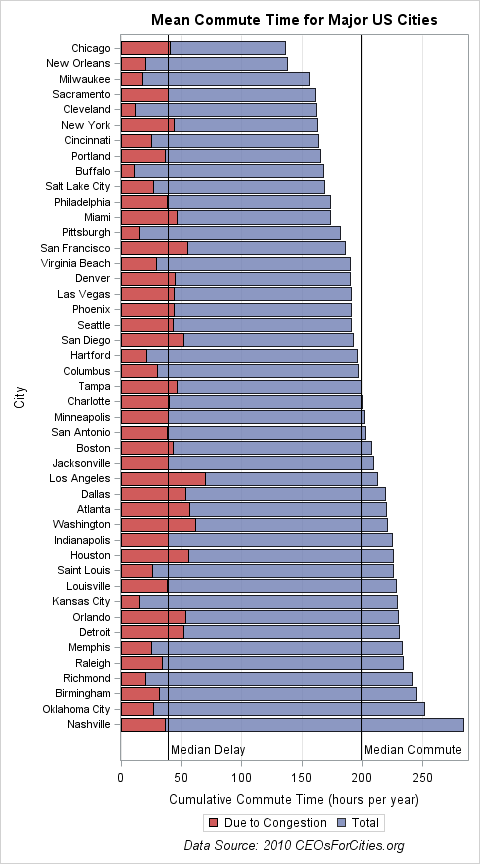The plot was created by using the SGPLOT procedure. The ODS GRAPHICS statement is used to set the dimensions of the plot so that all of the vertical labels show, and the vertical axis is offset to make room for the labels for the reference lines. Thanks to Sanjay Matange who told me about the NOSCALE option, which enables you to change the size of a graph without changing the size of the fonts.

```ods graphics / height=9in width=5in noscale; /* make a tall graph */ title "Mean Commute Time for Major US Cities"; footnote "Data Source: 2010 CEOsForCities.org";   proc sgplot data=traffic; hbar City / response=total_hours CategoryOrder=RespAsc transparency=0.2 name="total" legendlabel="Total"; hbar City / response=hours_of_delay name="delay" legendlabel="Due to Congestion"; keylegend "delay" "total"; xaxis grid label="Cumulative Commute Time (hours per year)"; yaxis ValueAttrs=(size=8) offsetmax=0.05; /* make room for labels */ refline 39 199 / axis=x label=("Median Delay" "Median Commute") lineattrs=(color=black) labelloc=inside; run;```

### Second graph: A scatter plot

Although the previous graph is probably the one that I would choose for a report that is intended for general readers, you can use a scatter plot to create a more statistical presentation of the same data.

In the bar chart, it is hard to compare cities. For example, I might want to compare the average commute and congestion in multiple cities. Because the cities might be far apart on the bar chart, it is difficult to compare them. An alternative visualization is to create a scatter plot of the commute time versus the time spent in traffic for the 45 cities. In the scatter plot, you can see that Nashville and Oklahoma City have moderate congestion, even though their mean commute times are large (presumably due to long commutes). In contrast, the congestion in cities such as Los Angeles, Washington, and Atlanta are evident.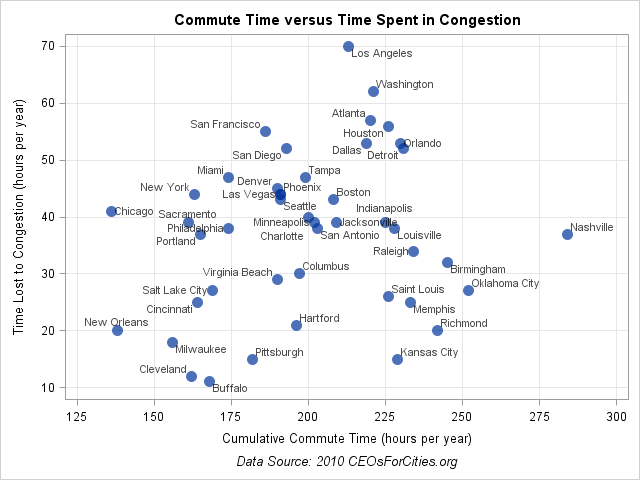The plot was created by using the SGPLOT procedure. The DATALABEL= option is used to label each marker by the name of the city.

```ods graphics / reset; /* reset graphs to default size */ title "Commute Time versus Time Spent in Congestion";   proc sgplot data=traffic; scatter x=total_hours y=hours_of_delay / MarkerAttrs=(size=12 symbol=CircleFilled) transparency=0.3 datalabel=City datalabelattrs=(size=8); xaxis grid label="Cumulative Commute Time (hours per year)" values=(125 to 300 by 25); yaxis grid label="Time Lost to Congestion (hours per year)"; run;```

For me, the surprising aspect of this data (and Rob's graph) is that the average commute times are more similar than I expected. Another interesting aspect is that my notion of "cities with bad commutes" seems to be based on congestion (the cities that are near the top of the scatter plot) rather than on long commutes (the cities to the right of the scatter plot).

What aspects of these data do you find interesting?The other day I was using PROC SGPLOT to create a box plot and I ran a program that was similar to the following:

```proc sgplot data=sashelp.cars; title "Box Plot: Category = Origin"; vbox Horsepower / category=origin; run;```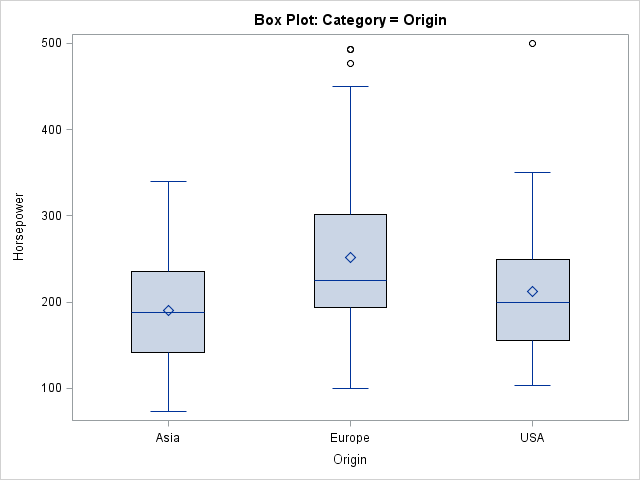An hour or so later I had a need for another box plot. Instead of copying the previous statements, I retyped in the PROC SGPLOT code. However, I wasn't paying attention and I typed GROUP= instead of CATEGORY= as the option to the VBOX statement:

```proc sgplot data=sashelp.cars ; title "Box Plot: Group = Origin"; vbox Horsepower / group=Origin; run;```When I saw the second graph, I noticed that it is more colorful and also has a legend instead of an axis with tick marks and labels. I wondered, "What is the difference between the CATEGORY= option and the GROUP= option?" I started making a set of notes, which I share in this article.

### The CATEGORY= option defines a categorical variable

The CATEGORY= syntax defines the discrete variable for the plot. As such, the values of the categorical variable appear as tick marks on an axis. All graphical elements have the same graphical styles, such as color, line pattern, marker shapes, and so forth.

The values of the categorical variable appear in alphabetical or numerical order, although some graphs support option for sorting the categories. For example, to order the categories in a bar chart in ascending or descending order, use the CATEGORYORDER= option in the VBAR statement.

For the box plot, specifying a categorical variable is optional and therefore the CATEGORY= option is specified after the slash (/). For most other plots (for example, the bar chart), the categorical variable is NOT optional, so the variable is specified before the slash.

As of SAS 9.3, the CATEGORY= variable can be numeric, which means that you can create box plots that are arranged on a continuous axis, as follows:
```proc sgplot data=sashelp.cars; title "Box Plot: Category = Cylinders, Linear Scale"; vbox horsepower / category=cylinders; /* SAS 9.3 example */ xaxis type=linear; run;```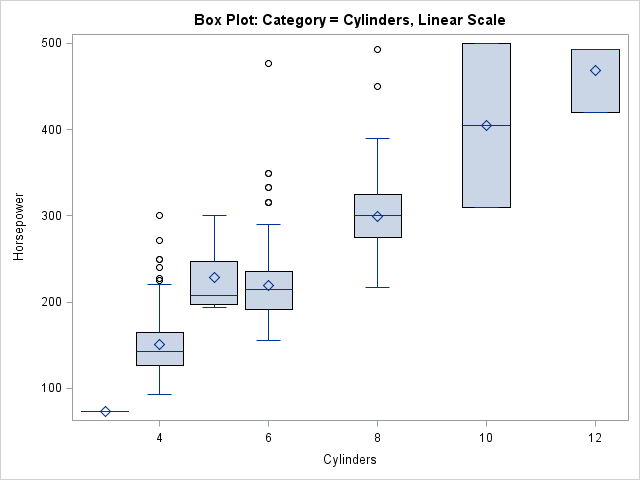In this graph, the XAXIS statement is used to specify that the number of cylinders should not be treated as a discrete (nominal) variable, but should be spaced according to their values on a continuous scale. (Notice the presence of vehicles with three and five cylinders in the data.) This can be a useful feature. For example, I use it to visualize the performance of algorithms. The X axis might be the number of variables in an analysis, and the box plot might represent the distribution of times for 10 runs of the algorithm.

The syntax for this "continuous variable box plot" is a bit contradictory: The CATEGORY= option specifies the X variable (which, from the syntax, you expect to be categorical!) and the TYPE=LINEAR option specifies that the X variable is continuous. However, this is a powerful syntax. It very useful for clinical trials data in which you plot the distribution of the response variable versus time for patients in experimental and control groups.

### The GROUP= option defines an auxiliary variable

The GROUP= option defines an auxiliary classification variable. I like to think of the GROUP= variable as defining an overlay of various "mini plots." In most cases, you get k mini plots for every one that you have without the GROUP= option, where k is the number of levels in the grouping variable. For example, in the line plot you get k overlaid lines, one for each group.

The main difference between the CATEGORY= and GROUP= options is that the GROUP= option results in graphical elements that have varying attributes. By default, each unique value of the grouping variable is drawn in a separate style element GraphData1 through GraphDatak. The association between graphical styles and the groups are shown in a legend.

The SGPLOT procedure supports many options that control the appearance of the grouped data. You can use the GROUPDISPLAY= option to specify that the grouped elements be clustered, overlaid, or (for bar charts) stacked. You can use the GROUPORDER= option to specify how you want the group elements to be ordered.

### Combining the two options

You can combine the two options to visualize a group variable nested within a categorical variable. The following statements create a graph that contains box plots for several types of vehicles, nested within the Origin variable:

```proc sgplot data=sashelp.cars; where Type in ('SUV' 'Truck' 'Sedan'); title "Box Plot: Category = Origin, Group = Type"; vbox horsepower / category=Origin Group=Type; run;```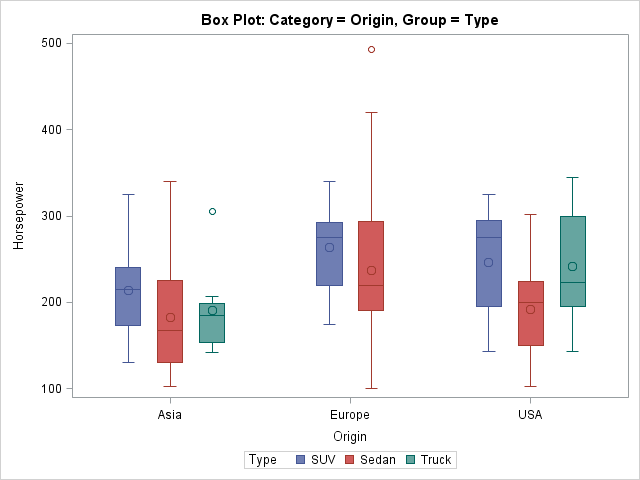The plot shows that the data set does not contain any trucks that are made in Europe. It also shows that sedans tend to have lower horsepower than SUVs, when you account for the Origin variable.

In summary, the VBOX (and HBOX) statements in the SGPLOT procedure support several options that arrange the boxes. The CATEGORY= option defines the variable to use for the X axis, whereas the GROUP= option defines an auxiliary discrete variable whose values and graphical attributes are displayed in a legend. You can use the options to visualize the distribution of one response variable with respect to one or two other variables.I've seen analyses of Fisher's iris data so often that sometimes I feel like I can smell the flowers' scent. However, yesterday I stumbled upon an analysis that I hadn't seen before.

The typical analysis is shown in the documentation for the CANDISC procedure in the SAS/STAT documentation. A (canonical) linear discriminant analysis (LDA) that uses four variables (SepalLength, SepalWidth, PetalLength, and PetalWidth) constructs hyperplanes that can almost separate the species. With an LDA, only three flowers are misclassified: one virginica is misclassified as a versicolor and two versicolor are misclassified as virginica. The following plot is from the SAS/STAT documentation and shows how the first canonical coordinate does a fantastic job of discriminating between the species.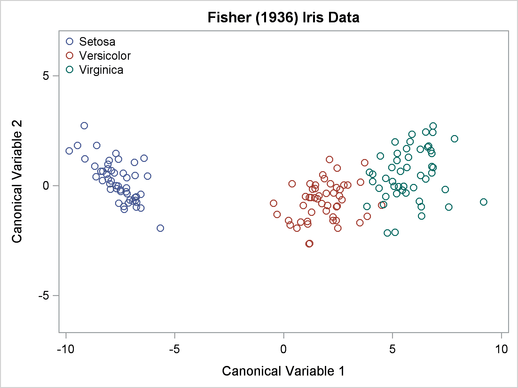I've always been impressed by this analysis. I consider it a remarkable example of the power of statistics to solve classification problems.

Yesterday I saw a Web page from StatLab Heidelberg at the Institute for Applied Mathematics, Universität Heidelberg. The author points out that the area of the petals and sepals do a much better job of discriminating between the various species. In fact, they point out that you do not even need to use discriminant analysis to obtain a result that is almost as good as LDA!

The following DATA step constructs the area of the sepals and petals, assuming that they are nearly rectangular. You can then plot the new variables:

```data iris; set Sashelp.Iris; SepalArea = SepalLength * SepalWidth; PetalArea = PetalLength * PetalWidth; run;   proc sgplot data=iris; title "Discriminant Analysis of Iris Data Using Area"; scatter x=SepalArea y=PetalArea / group=Species; refline 150 750 / axis=y; /* add "dividing lines" */ run;```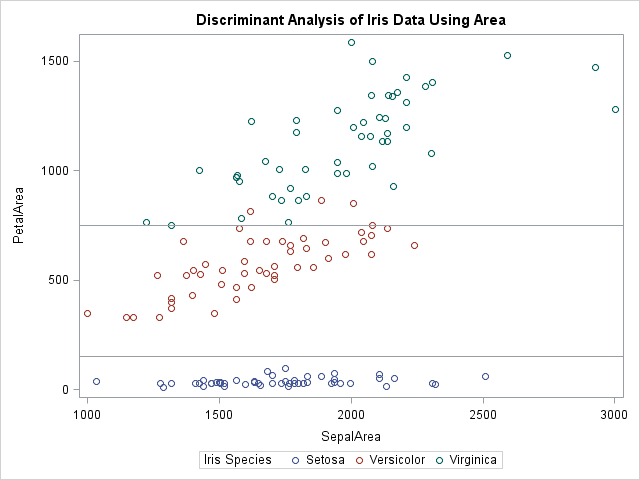The single variable, PetalArea, does nearly as good a job at classifying the iris species as linear discriminant analysis. In the scatter plot, you can draw horizontal lines that nearly separate the species. The lines that are drawn misclassify only four versicolor as virginica. That's an amazing result for using a single, easily constructed, variable, which has the additional advantage of having physical significance.

I have seen a similar idea used in the discriminant and cluster analysis of the Sashelp.Fish data set. For the fish, it is common to transform weight by a cube-root transformation in order to obtain a variable that is comparable to the heights, widths, and lengths of the fish. However, until now I had never thought about using the area of the iris flower parts.

The iris quadratic transformation is impressive because of its simplicity. I wonder when this was first noticed. Can anyone provide a reference that precedes the 2005 date on the StatLab web page? An internet search located one instance of this transformation in the book Data Clustering and Pattern Recognition, which was printed in Chinese, but I cannot determine who originated the idea.To celebrate special occasions like Father's Day, I like to relax with a cup of coffee and read the newspaper. When I looked at the weather page, I was astonished by the seeming uniformity of temperatures across the contiguous US. The weather map in my newspaper was almost entirely yellow and orange, which told me that the midday temperatures were predicted to have extremely small variation. It looked like the range of temperatures might vary by less than 30 degrees Fahrenheit (17 degrees Celsius), with most temperatures in the 70's and 80's.

To me this seemed like an extremely small variation, so that afternoon I downloaded the actual midday temperatures from Weather.com, which are shown below: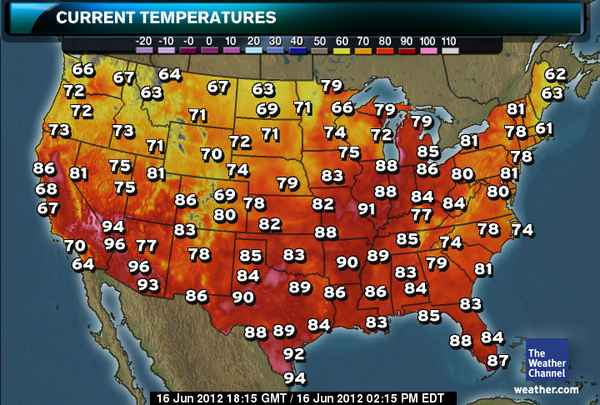The Weather.com map uses a different color scale than my local paper. Nevertheless I quickly estimated that most of the temperatures were between 62 F and 92 F, with just a few hot spots in southern Texas, Arizona, and Nevada. The statistician in me wondered, what is the record for the "most uniform" temperatures at midday in the contiguous US? I'll bet that someone can automate the downloading of temperatures for standard US locations and answer the question. I'd love to see a graph of "temperature variation vs. date" for, say, the last ten or twenty years.

I don't know of a source for temperature data, but I figured that I might as well do SOME statistics to celebrate Father's Day weekend! I enlisted my youngest child to assist me in typing in the temperatures. (Yes, it was a father/daughter bonding moment.) I ran the following univariate analysis of the temperatures:

```data Temps06162012; input Temperature @@; datalines; 66 72 72 73 86 81 68 67 70 64 64 67 63 73 71 75 81 75 94 96 77 96 93 67 71 72 70 74 69 86 80 83 78 86 63 69 71 71 79 78 82 83 85 84 89 90 88 89 92 94 79 66 79 74 72 75 88 83 88 82 91 88 89 90 86 86 83 84 79 81 85 86 80 84 84 77 85 74 79 83 84 85 62 63 81 61 78 78 81 80 74 78 81 83 84 88 87 ;   proc univariate data=Temps06162012; var Temperature; histogram Temperature; run;```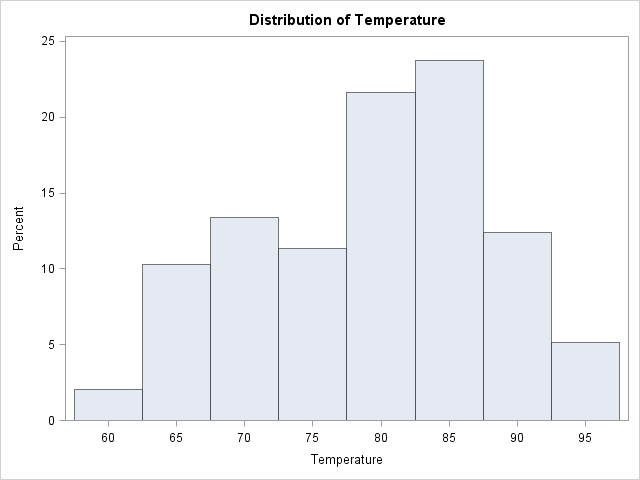The histogram and the accompanying tabular output told me that the mean temperature was a comfortable 79 degrees F, and that the standard deviations of temperatures was a paltry 8.56 degrees F. It was, indeed, a beautiful weekend for celebrating Father's Day.

Can anyone find a date for which the temperatures exhibited less variation? I'd love to see the analysis.The other day I encountered an article in the SAS Knowledge Base that shows how to write a macro that "returns the variable name that contains the maximum or minimum value across an observation." Some people might say that the macro is "clever." I say it is complicated. This is a simple problem; it deserves a simple solution.

This is one of those situations where a SAS/IML implementation is simpler and cleaner than a macro/DATA step solution. The following DATA step creates the data that are used in the SAS Knowledge Base article:

```/* Data for Sample 46471: Return the variable name that contains the max or min value across an observation */ data one; input a b c d e; cards; 1 3 12 6 15 34 583 294 493 2 ; run;```

By inspection, the minimum value in the first row is 1, which occurs for the variable A. In the second row, the minimum value is 2, which occurs for the variable E.

To find the variable for each row that contains the minimum value for that row, you can use the index minimum subscript reduction operator, which has the symbol >:<. The subscript reduction operators are a little-known part of the SAS/IML language, but they can be very useful. The following SAS/IML program begins by reading all numerical variables into a matrix, X. The subscript reduction operator then computes a column vector whose ith element is the column for which the ith row of X is minimal. You can use this column vector as an index into the names of the columns of X.

```/* For each row, find the variable name corresponding to the minimum value */ proc iml; use one; read all var _NUM_ into X[colname=VarNames]; close one;   idxMin = X[, >:<]; /* find columns for min of each row */ varMin = varNames[idxMin]; /* corresponding var names */ print idxMin varMin;```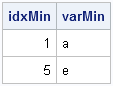Yes, those two statements compute the same quantity as the complicated macro. And, if you are willing to nest the statements, you can combine them into a single statement:
varNames[X[, >:<]].

Finding the maximum value for each row is no more difficult: simply use the <:> subscript reduction operator.

The macro featured in the Knowledge Base article includes an option to compute with specified variables, rather than all numerical variables. That, too, is easily accomplished. For example, the following statements find the variable that has the largest value among the A, B, and C variables:

```varNames = {a b c}; use one; read all var varNames into X; close one;   idxMax = X[,<:>]; varMax = varNames[idxMax]; print idxMax varMax;```My next post will discuss subscript reduction operators in further details.

To my readers who are SQL experts: Is there a simple way to solve this problem by using PROC SQL? Leave a comment.I want to create a vector as follows. Suppose there are two given vectors x=[A B C] and f=[1 2 3]. Here f indicates the frequency vector. I hope to generate a vector c=[A B B C C C]. I am trying to use the REPEAT function in the SAS/IML, language but there is always something wrong. Can you help me?

This is probably a good time to remind everyone about the SAS/IML Community (formerly known as a Discussion Forum). You can post your SAS/IML questions there 24 hours a day. That is always a better plan than making a personal appeal to me, because I receive dozens of questions like this every month, and there is no way that I can personally reply. There are lots of experienced SAS/IML experts out there, so please use the SAS/IML Community to tap into that knowledge.

That said, I think the answer to this reader's question makes an interesting example of statistical programming with SAS/IML software. It is trivial to solve this in the DATA step (see the end of this article), but how might you solve it in the SAS/IML language? If you'd like to try to solve this problem yourself, stop reading here. Spoilers ahead!

### Create a new vector that duplicates frequencies

The goal is to write a function that duplicates or "expands" data that have a frequency variable. The important function to use for this task is the CUSUM function, which computes the cumulative frequencies. Let's look at a simple example and apply the CUSUM function to the frequency vector:

```proc iml; values={A,B,C,E}; freq = {2,1,3,4}; cumfreq = cusum(freq); print values freq cumfreq;```As shown in the output, the cumfreq variable contains the indices for the expanded data. The expanded data will be a vector that contains 10 elements. The first data value (A) repeats twice (the freq value), so it repeats until element 2 (the cumfreq value) in the expanded vector. The second category fills element 3. The next category repeats 3 times, so it occupies up through element 6 in the expanded vector. The last category repeats until element 10. The following DO loop specifies each data value and the indices of the expanded vector that it should occupy:

```print (values)[label="value"] (1:cumFreq)[label="Indices"]; do i = 2 to nrow(values); bIdx = 1 + cumFreq[i-1]; /* begin index */ eIdx = cumFreq[i]; /* end index */ value = values[i]; print value (bIdx:eIdx)[label="Indices"]; end;```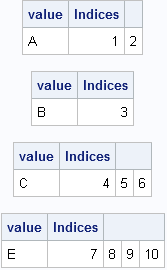The output shows that we have all the information we need to allocate a vector of length 10 and fill it with the data values, where the ith value is repeated freq[i] times. The key, it turns out, is to use the CUSUM function to find the indices that correspond to the each data value.

### A module to compute the expanded data

In SAS procedures that support a FREQ statement, the frequency values must be positive integers. If the frequency value is missing or is a nonpositive value, the corresponding data value is excluded from the analysis. It is easy to add that same feature to a module that takes a vector of values and a vector of frequencies and returns a vector that contains the data in expanded form. This is implemented in the following SAS/IML module, which allocates the result vector with the first data value in order to avoid handling the first element outside of the DO loop:

```start expandFreq(_x, _freq); /* Optional: handle nonpositive and fractional frequencies */ idx = loc(_freq > 0); /* trick: in SAS this also handles missing alues */ if ncol(idx)=0 then return (.); x = _x[idx]; freq = round( _freq[idx] );   /* all frequencies are now positive integers */ cumfreq = cusum(freq);   /* Initialize result with x to get correct char/num type */ N = nrow(x); expand = j(cumfreq[N], 1, x); /* useful trick */   do i = 2 to N; bIdx = 1 + cumFreq[i-1]; /* begin index */ eIdx = cumFreq[i]; /* end index */ expand[bIdx:eIdx] = x[i];/* you could use the REPEAT function here */ end; return ( expand ); finish;   /* test the module */ values={A,B,C,D,E,F}; freq = {2,1,3,0,4,.}; /* include nonpositive and missing frequencies */ y = expandFreq(values, freq); print values freq y;```Notice that you don't actually need to use the REPEAT function because SAS/IML is happy to assign a scalar value into a vector. The scalar is automatically repeated as often as needed in order to fill the vector.

### A DATA step solution

As indicated at the beginning of this post, the DATA step solution is quite simple: merely use the OUTPUT statement in a loop, as shown in the following example:

```data Orig; input x \$ Freq; datalines; A 2 B 1 C 3 D 0 E 4 F . ; run;   /* expand original data by frequency variable */ data Expand; keep x; set Orig; if Freq<1 then delete; do i = 1 to int(Freq); output; end; run; proc print data=Expand; run;```

The output data set contains the same data as the y vector in the SAS/IML program.SAS software provides many run-time functions that you can call from your SAS/IML or DATA step programs. The SAS/IML language has several hundred built-in statistical functions, and Base SAS software contains hundreds more. However, it is common for statistical programmers to extend the run-time library to include special user-defined functions.

In a previous blog post I discussed two different ways to apply a log transformation when your data might contain missing values and negative values. I'll use the log transformation example to show how to define and call user-defined functions in SAS/IML software and in Base SAS software.

### A "safe" log transformation in the SAS/IML language

In the SAS/IML language, it is easy to write user-defined functions (called modules) that extend the functionality of the language. If you need a function that safely takes the natural logarithm and handles missing and negative values, you can easily use the ideas from my previous blog post to create the following SAS/IML function:

```proc iml; /* if Y>0, return the natural log of Y otherwise return a missing value */ start SafeLog(Y); logY = j(nrow(Y),ncol(Y),.); /* allocate missing */ idx = loc(Y > 0); /* find indices where Y > 0 */ if ncol(idx) > 0 then logY[idx] = log(Y[idx]); return(logY); finish;   Y = {-3,1,2,.,5,10,100}; LogY = SafeLog(Y); print Y LogY;```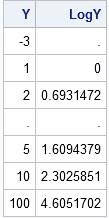The program is explained in my previous post, but essentially it allocates a vector of missing values and then computes the logarithm for the positive data values. The START and FINISH statements are used to define the SafeLog function, which you can then call on a vector or matrix of values.

In this example, the function is defined only for the current PROC IML session. However, you can store the function and load it later if you want to reuse it.

### Defining a "safe" log transformation by using PROC FCMP

You can also extend the Base SAS library of run-time functions. The FCMP procedure enables you to define your own functions that can be called from the DATA step and from other SAS procedures. (The MCMC procedure has an example of calling a user-defined function from a SAS/STAT procedure.) If you have never used the FCMP procedure before, I recommend Peter Eberhardt's 2009 paper on defining functions in PROC FCMP. For a more comprehensive treatment, see Jason Secosky's 2007 paper.

Technically, you don't need to do anything special in the DATA step if you want a SAS missing value to represent the logarithm of a negative number: the DATA step does this automatically. However, the DATA step also generates some scary-looking notes in the SAS LOG:

```NOTE: Invalid argument to function LOG at line 72 column 5.
RULE:      ----+----1----+----2----+----3----+----4----+----5----+----6----+----7----+----8----+
74         -3 1 2 . 5 10 100
x=-3 y=. _ERROR_=1 _N_=1
NOTE: Missing values were generated as a result of performing an operation on missing values.
NOTE: Mathematical operations could not be performed at the following places. The results of
the operations have been set to missing values.
```

I prefer my programs to run with a clean, healthy-looking SAS LOG, so I will use PROC FCMP to define a SafeLog function that has the same behavior (and name!) as my SAS/IML function:

```proc fcmp outlib=work.funcs.MathFuncs; function SafeLog(y); if y>0 then return( log(y) ); return( . ); endsub; quit;```

The function returns a missing value for nonpositive and missing values. The definition of the function is stored in a data set named WORK.FUNCS, which will vanish when you exit SAS. However, you can create the definition in a permanent location if you want to call the function in a later SAS session.

In order to call the function from the DATA step, use the CMPLIB= option, as shown in the following example:

```options cmplib=work.funcs; /* define location of SafeLog function */ data A; input x @@; y = SafeLog(x); /* test the function */ datalines; -3 1 2 . 5 10 100 ; run;```

The result is not shown, but it is identical to the output from the SAS/IML program.

You might not have need for the SafeLog function, but it is very useful to know how to define user-defined functions in SAS/IML software and in Base SAS software. SAS/IML modules and PROC FCMP functions make it easy to extend the built-in functionality of SAS software.